Advances in Wireless Communications and Networks
Volume 2, Issue 1, September 2016, Pages: 11-14

An Improved Redundant Residue Number System Based Error Detection and Correction Scheme for the Moduli Set {22n + 1, 2n+1 + 1, 2n+1 – 1, 2n + 1, 2n}

1Department of Computer Science University for Development Studies, Navrongo, Ghana

2Department of Computer Science College of Information and Communication Tech, Kwara State University, Malete, Nigeria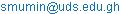(S. Abdul-Mumin)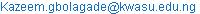(K. A. Gbolagade)

Salifu Abdul-Mumin, Kazeem Alagbe Gbolagade. An Improved Redundant Residue Number System Based Error Detection and Correction Scheme for the Moduli Set {22n + 1, 2n+1 + 1, 2n+1 – 1, 2n + 1, 2n}. Advances in Wireless Communications and Networks. Vol. 2, No. 1, 2016, pp. 11-14. doi: 10.11648/j.awcn.20160201.12

Received: October 6, 2016; Accepted: November 4, 2016; Published: December 5, 2016

Abstract: Data integrity has tremendous effects on any data communication system’s performance. Communication systems are probabilistic in nature and may fail due to errors generated during the transmission process. These errors are generated due to various factors as noise, heat and other disturbance from neighboring systems. In this paper, an error detection and correction scheme based on redundant residue number system is presented. A novel 5-moduli set {22n + 1, 2n+1 + 1, 2n+1 – 1, 2n + 1, 2n}, for n even, is utilized. The first three moduli is the information moduli set while the last two moduli are redundant that are used for the error detection and correction. Consequently, an error detection and correction algorithm is proposed. The number of iterations in the error correction scheme has been tremendously reduced which in turn reduces the design complexity and also decreases the propagation delay.

Keywords: Data Communication, Error Detection and Correction, Redundant Residue Number System

Contents

1. Introduction

Properties of Redundant Residue Number System have been used for detecting and correcting potential errors during the transmission process. Similar scheme was presented in . Our proposed system is very straight forward and it is not complex to implement. The number of iterations in the correction scheme have been tremendously reduced which in turn reduces the design complexity and decreases the propagation delay.

In this paper, an error detection and correction scheme based on redundant residue number system is presented. A novel 5-moduli set {22n + 1, 2n+1 + 1, 2n+1 – 1, 2n + 1, 2n}, for n even, is utilized. The first three moduli is the information moduli set while the last two moduli are redundant that are used for the error detection and correction. Consequently, an error detection and correction algorithm is proposed. The number of iterations in the error correction scheme has been tremendously reduced which in turn reduces the design complexity and also decreases the propagation delay.

The rest of the paper is organized as follows: Section A discusses the background and some terminologies used, Section B gave the basic concepts of Redundant Residue Number system, Section C showed RRNS is useful for error detection and correction, Section D demonstrated the proposed algorithm, Section E presented a performance evaluation with the state of art, and the paper is concluded in Section F.

2. Background and Terminologies

In this section, the various key terms and the terminologies used in this work is discussed. During the ancient study of number system, computer scientist rediscovered RNS, who sought to exploit their good properties in the implementation of fast arithmetic and fault-tolerant computing. This 1700-year-old number system has been attracting a great deal of attention recently. Digital systems structured into residue arithmetic units may play an important role in ultra-speed, dedicated, real-time systems that support pure parallel processing of integer-valued data. It is a "carry free" system that performs addition, subtraction, and multiplication as concurrent (parallel) operations, sidestepping one of the principal arithmetic delays managing carry information . The residue representations carry no weight-information hence an error in any digit-position in a given representation does not affect other digit-positions.  There are however problems with RNS that have limited their practical implementation; these are sign detection, magnitude comparison, overflow detection, division and other complex arithmetic operations like square root, exponentiation etc.

RNS is defined in terms of a set of relatively prime moduli. If P denotes the moduli set, thenfor i ≠ j. Any integer X in the range [0, M) where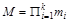(1)

can be uniquely and unambiguously represented by the residue sequence: X↔ (x1, x2 xk) where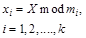(2)

Is the residue modulus mi of X. The range [0, M) is called dynamic range or the legitimate range of X.  .

Given a residue sequence (x1, x2, xk), the corresponding integer X in [0, M) can be uniquely recovered from the k residues using the Chinese Remainder Theorem (CRT). According to the CRT, for any given k-tuple (x1, x2, xk), where 0 ≤ xi < mi; i = 1, 2,.k, then the value of X can be found from the residue using;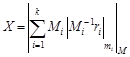(3)

Where from equation (1),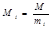(4)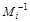is referred to as the multiplicative inverse corresponding to mi. .

3. Redundant Residue Number System (RRNS)

An RRNS is defined as a chosen RNS with additional redundant moduli. Each redundant modulus is generally greater than any of the moduli of the chosen moduli set. Assuming the standard RNS consists of the moduli set of {m1, m2, mk}, the corresponding RRNS consists of a moduli set of {m1, m2, mk, mk+2r} (r ≥ 1)   .

The RRNS has error detection and correction capability. By using 2r (r ≥ 1) redundant moduli, r errors can be detected and corrected .

The residues in RNS will serve as several channels for data communication (fault tolerant; each digit result is completely independent).

4. Rrns as Used in Error Detection and Correction

RNS are also useful in error detection and correction. This apparent, given the independence of digits in a residue-number representation: an error in one digit does not corrupt any other digits. In general, the use of redundant moduli, that is extra moduli that play no role in determining the dynamic range, facilitates both error detection and correction. The error detection and correction is done at the reverse converse of the general RNS processor. Therefore the algorithm is incorporated in a reverse converter. But even without redundant moduli, fault-tolerance is possible, since computation can still continue after the isolation of faulty digit-positions, provided that a smaller dynamic range is acceptable .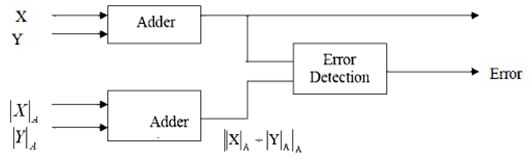Figure 1. Circuit Error Detection .

5. Proposed Algorithm

The algorithm has been proposed using the moduli set: {22n + 1, 2n+1 + 1, 2n+1 – 1, 2n + 1, 2n} for n even Where {2n+1 – 1, 2n + 1, 2n} is the information moduli set and {22n + 1, 2n+1 + 1} is the redundant moduli set.

To illustrate the following detection and correction mechanism, consider n = 2. The moduli set will be (17, 9, 7, 5, 4) where (7, 5, 4) is the information moduli set and (17, 9) is the redundant moduli set.

If a message X = 21 is to be transmitted using the moduli set above, the transmitted residues will be given as21 = (0, 1, 1)RNS (7 |5 |4). Let (0, 1, 1) = (x2, x1, x0). Assuming there is an error in the transmission channel due to either noise or other transmission impairments, and x0 is changed to 3which resulted in the received residue to be like (0, 1, 3).

Using the mixed-radix conversion, X is obtained as follows:

Let the corresponding mixed-radix values, be (z2, z1, z0). But x0 = z0 = 3

Subtracting 3 and dividing by 4, will give the following

(4, 3, 0)/4 = (4, 3, 0) * (2, 4, -) = (1, 2, -). Therefore z1 = 2

Subtracting 2 and dividing by 5, will give the following

(6, 0, -)/5 = (6, 0, -) * (3, -, -) = (4, -, -). Therefore z2 = 4

Therefore (0. 1, 3)RNS(7 | 5 | 4) = (4, 2, 3)MRS((7 | 5 | 4)

X = 4 * (5 * 4) + 2 * + 3 = 91

For detection of the error, take 91mod9 = 1 which is not 3 as required. The second residue can be further found by the second redundant modulus by 91mod17 = 6 which is not 4. It can therefore be concluded that an error has occurred in the transmission of X = 21.

For the correction of the error, the modulus that generated the error must be identified. The error was either generated by m2, or m1 or m0. So a test on each of the information moduli with the two redundant moduli will present the following:

(m4, m3, m0), (m4, m3, m1) and (m4, m3, m2)

For (m4, m3, m0), X =21 = (4, 3, 3) RNS (17 | 9 | 4).

Note: The residue, 3 is replaced with the residue, 1due to the error generated from m0 during the transmission. Using the MRC, X can be obtained as follow:

X0 = z0 = 3. So subtracting 3 and diving 4, will lead to the following: (1, 0, 0) /4 = (1, 0, 0) * (13, 7, -) = (13, 0, -). Therefore z1 = 0. So subtracting 0 and dividing 9, will present,

(13, 0,-) / 9 = (13, 0, -) * (2, - -) = (9, -, -). Therefore z2 = 9

Hence,

(4, 3, 3) RNS (17 | 9 | 4) = (9, 0, 3)MRS(17 | 9 | 4)

X = 4 * (9 * 4) + 0 * + 3 = 147

For (m4, m3, m1), X =21 = (4, 3, 1)RNS(17 | 9 | 5). Using the MRC, X can be obtained as follows:

X0 = z0 = 1. Subtracting 1 and dividing by 5, will result to

(3, 2, 0) / 5 = (3, 2, 0) * (7, 2, -) = (4, 4, -). Therefore z1 = 4. Subtracting 4 and dividing 9,

(0, 0, -) / 9 = (0, 0, -) * (2, -, -) = (0, -, -). Therefore z2 = 0

Therefore (4, 3, 1)RNS(17 | 9 | 5) = (0, 4, 1)MRS(17 | 9 | 5)

X = 0 * (9 * 5) + 4* + 1 = 21

For (m4, m3, m2), X =21 = (4, 3, 0)RNS(17 | 9 | 7). Using the MRC, X can be obtained as follows:

X0 = Z0 = 0. Subtracting 0 and dividing 7, will lead to the following; (4, 3, 0) / 7 = (4, 3, 0) * (5, 4, -) = (3, 3, -). Implies z1 = 3. Subtracting 3 and dividing 9,

(0, 0, -) / 9 = (0, 0, -) * (2, -, -) = (0, -, -). Implies z2 = 0

Therefore (4, 3, 0)RNS(17 | 9 | 7) = (0, 3, 0)MRS(17 | 9 | 7)

X = 0 * (9 * 7) + 3* + 0 = 21

It can henceforth be observe that the error was generated by m0. It is concluded that the correct result is 21 and that there was an error in x0, which can be corrected by computing.

X0 = 21 (mod 4) = l

6. Performance Analysis

Results of this paper are compared to the results in  where the number of iterations performed in order to correct a single error with 5- moduli set is ten; that is a complete recombination. This has a total of k (k-1)/2 number of iterations/recombination, where k is the number of moduli set. This means the correction algorithm in RRNS with k moduli set has an asymptotic complexity in the order of O (k2). In this paper however, only three combinations or iterations are required. This has a total of (k + 1)/2 for k being odd and (k + 2)/2 for k being even number of iterations/recombination. Therefore the correction algorithm in RRNS with k moduli set has an asymptotic complexity in the order of O (k). Hence, given the same conditions for the error detection and correction at the reverse conversion, the delay in this scheme is expected to be reduced to about 70%. The amount of memory also needed to design this architecture is expected to reduce significantly.

7. Conclusion

Errors are inevitable in data communication due to various factors like noise, heat, interference in the communication circuits. Thus, there is need for fast error detection and correction schemes. This proposed scheme detects and corrects a single error using RRNS. Five-length moduli set have been proposed where the first three moduli set is the information moduli and the last two is the redundant moduli for error detection and correction. The proposed scheme have only three recombination or iterations to detect and correct a transmission error whiles the state of the will need ten recombination or iterations. As such, the proposed scheme is very simple and straightforward and the time it will take to correct an error is relatively lesser than other detection and correction schemes.

References

1. D.K.Sharma, A. Mishra & Rajiv Saxena, Analog and Digital Modulation Techniques: An Overview TECHNIA- International Journal of Computing Science and Communication Technologies, VOL. 3, NO. 1, July 2010. (ISSN 0974-3375)
2. Vik Tor Goh and Mohammad Umar Siddiqi, Multiple Error Detection and Correction Based on Redundant Residue Number Systems, IEEE TRANSACTIONS ON COMMUNICATIONS, VOL. 56, NO. 3, MARCH 2008
3. M. Roshanzadeh, A. Ghaffari and S. Saqaeeyan, Using Residue Number Systems for Improving QoS and Error Detection & Correction in Wireless Sensor Networks,Communication Software and Networks (ICCSN),May2011 IEEE 3rd International Conference onPage: 1-5
4. Salifu Abdul-Mumin, Detection of Man-in-the-Middle attack in Computer Network i-manager’s Journal on Communication Engineering and Systems, Vol. 2 No. 1 November 2012 - January 2013
5. Fred J. Taylor, Residue Arithmetic: A Tutorial with Examples,IEEE Computer Society PressLos Alamitos, CA, USAVolume 17 Issue 5, May 1984 Pages 50-62
6. K. A. Gbolagade, An Efficient MRC based RNS-to-Binary Converter for the moduli set, {22n+1-1, 2n, 22n-1}, AIMS SA, 2011
7. Amir Sabbagh Molahosseini and Keivan Navi, New Arithmetic Residue to Binary Converters International Journal of Computer Sciences and Engineering Systems, Vol.1, No.4, October 2007 CSES International©2007 ISSN 0973-4406
8. Duc-Minh Pham, A. B. Premkumar and A. S. Madhukumar, Error Detection and Correction in Communication Channels Using Inverse Gray RSNS Code, IEE Transactions on Communications59(4):975-986 April 2011
9. Jenn-Dong Sun and Hari Krishna, Fast Algorithm for Multiple Errors Detection and Correction in Redundant Residue Number System Journal of Circuit, Systems and Signal Processing December Volume 12, IssueIssue 4,pp 503-531, 1993
10. Hari Krisna, Kuo-Yu Lin, and Jenn-Dong Sun, A coding Theory Approach to Error Control in Redundant Residue Number Systems- Part I: Theory and Single Error Correction, IEEE Transactions on Circuits and Systems II: Analog and Digital Signal Processing Vol 39 issue 1 pp 8-17 Jan 1992

 Contents 1. 2. 3. 4. 5. 6. 7.
Article ToolsAbstractPDF(230K)